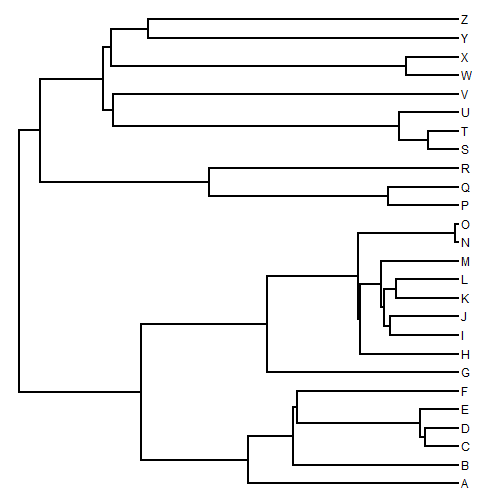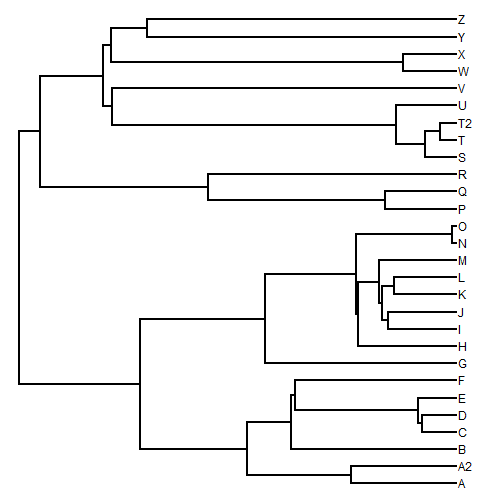## Saturday, August 29, 2015

### Adding a tip to a sister taxon on the tree

Here's a quick primer on how to attach a tip not in our tree to it's putative sister taxon half-way along the terminal edge length to its sister taxon.

``````## load phytools & simulate
library(phytools)
tree<-pbtree(n=26,tip.label=LETTERS)
plotTree(tree)
````````````## add tip "A2" to sister taxon "A"
tip<-"A2"
sister<-"A"
tree<-bind.tip(tree,tip,where=which(tree\$tip.label==sister),
position=0.5*tree\$edge.length[which(tree\$edge[,2]==
which(tree\$tip.label==sister))])
## add tip "T2" to "T"
tip<-"T2"
sister<-"T"
tree<-bind.tip(tree,tip,where=which(tree\$tip.label==sister),
position=0.5*tree\$edge.length[which(tree\$edge[,2]==
which(tree\$tip.label==sister))])
plotTree(tree)
``````This works with ultrametric trees. If our trees are not ultrametric, then we also have to include the terminal edge length that we want to have for our tip to be added in each case.

OK, we've done it!

1.Worked like a charm. Thanks so much!!

2.This comment has been removed by the author.

3.Sorry to bother you but how can we create a function to add several tips to several sister taxa? I tried this but cannot make it work. Thanks for the help.

tip<- c("A1", "B1")
sister<- c("A", "B")
for (i in 1:length(tip)) tree<- bind.tip(tree,tip[i], where=which(tree\$tip.label==sister[i]), position=0.5*tree\$edge.length[which(tree\$edge[,2]==which (tree\$tip.label==sister[i]))])

4.Thanks Liam!

5.This comment has been removed by the author.

Note: due to the very large amount of spam, all comments are now automatically submitted for moderation.